# Go Math Grade 4 Answer Key Homework FL Chapter 10 Two-Dimensional Figures Review/Test

## Go Math Grade 4 Answer Key Homework FL Chapter 10 Two-Dimensional Figures Review/Test

Practice number of questions in Go Math Grade 4 Answer Key Homework FL Chapter 10 Two-Dimensional Figures Review/Test. You can test your knowledge with the help of the Review Test. Practice the questions and check the answers in Go Math Grade 4 Answer Key Homework FL Chapter 10 Two-Dimensional Figures Review/Test. Tap the below links and download the answers.

### Review/Test – Page No. 411

Choose the best term from the box to complete the sentence.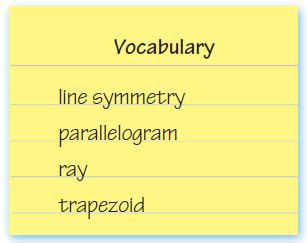Question 1.
A ____________ is a quadrilateral with exactly one pair of parallel sides.
________

A Trapezoid is a quadrilateral with exactly one pair of parallel sides.

Question 2.
A shape has _____________ if it can be folded about a line so that its two parts match exactly.
________

A shape has Line Symmetry if it can be folded about a line so that its two parts match exactly.

Question 3.
A ____________ has one endpoint and continues without end in one direction.
________

A Ray has one endpoint and continues without end in one direction.

Tell if the blue line appears to be a line of symmetry.
Write yes or no.

Question 4.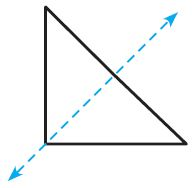_____

Explanation:
The line of symmetry divides a shape into two parts that are the same size and shape.

Question 5.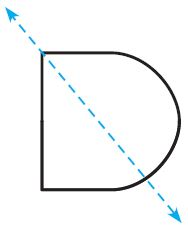_____

Explanation:
The line of symmetry divides a shape into two parts that are not of the same size and shape.

Question 6.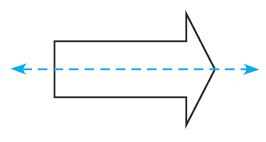_____

Explanation:
The line of symmetry divides a shape into two parts that are the same size and shape.

Use Figure A for 7–9.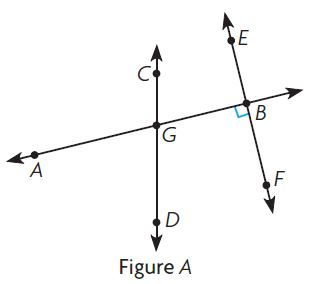Question 7.
Name a pair of perpendicular lines.
Type below:
________

Answer: $$\overleftrightarrow { AB }$$ and $$\overleftrightarrow { BF }$$

Explanation:
The pair of perpendicular lines in the above figure is $$\overleftrightarrow { AB }$$ and $$\overleftrightarrow { BF }$$

Question 8.
Name a pair of intersecting lines that are not perpendicular.
Type below:
________

Answer: $$\overleftrightarrow { AG }$$ and $$\overleftrightarrow { GD }$$

Explanation:
The pair of intersecting lines that are not perpendicular lines in the above figure is $$\overleftrightarrow { AG }$$ and $$\overleftrightarrow { GD }$$.

Question 9.
Classify ∠AGD. Write acute, right, or obtuse.
________

Explanation:
The angle ∠AGD is less than 90° thus the figure is an acute angle.

### Review/Test – Page No. 412

Question 10.
Which describes the shape?Options:
a. zero lines of symmetry
b. 1 line of symmetry
c. 2 lines of symmetry
d. more than 2 lines of symmetry

Explanation:
The trapezoid has only a vertical line of symmetry.
Thus the above shape is a trapezoid and it has one line of symmetry.
Thus the correct answer is option b.

Question 11.

Which figure does not have two pairs of parallel sides?
Options:
a. parallelogram
b. trapezoid
c. rhombus
d. square

Explanation:
The parallelogram has no lines of symmetry.
Thus the correct answer is option a.

Question 12.
How many right angles can be in an obtuse triangle?
Options:
a. 0
b. 1
c. 2
d. 3

Explanation:
An obtuse triangle is a triangle with one obtuse angle greater than 90°.
Thus the correct answer is option b.

Question 13.
Which is the correct label for a right angle in the figure?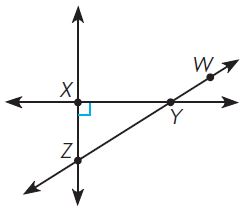Options:
a. ∠XYZ
b. ∠XYW
c. ∠ZXY
d. ∠ZYX

Explanation:
The right angle is at point X. So, the correct label for a right angle in the figure is ∠ZXY.
Thus the correct answer is option c.

Question 14.
Which of the following letters of the alphabet has line symmetry?
Options:
a. S
b. F
c. H
d. N

Explanation:
The line of symmetry divides a shape H into two parts that are the same size and shape.
Thus the correct answer is option c.

### Review/Test – Page No. 413

Question 15.
Which statement is true?
Options:
a. A trapezoid can never have a right angle.
b. A parallelogram can never have a right angle.
c. A rhombus is a type of trapezoid.
d. A square is a type of parallelogram.

Answer: A square is a type of parallelogram.
Square – A parallelogram with four sides of equal length and angles of equal size.
Thus the correct answer is option d.

Question 16.
Which lines appear parallel?
Options:
a.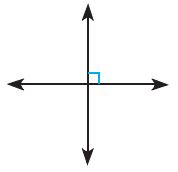b.c.d.Answer:Explanation:It has 2 intersecting lines so the lines are not parallel.The lines are not intersecting. So the lines are parallel.
Thus the correct answer is option b.

Question 17.
Norris drew the pattern below.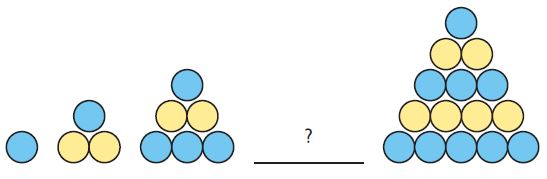Which is the missing figure in the pattern?
Options:
a.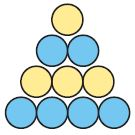b.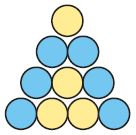c.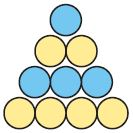d.Explanation:
By seeing the above pattern we can guess the missing figure.
Thus the correct answer is option a.

### Review/Test – Page No. 414

Describe a pattern. Write a rule using numbers to find the number of squares in any figure in the pattern.

Question 18.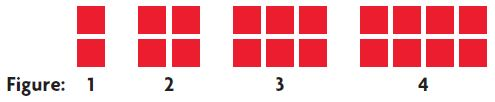Type below:
________

Question 19.
Classify the figure as many ways as possible. Write quadrilateral, trapezoid, parallelogram, rhombus, rectangle, or square.________

The above figure is a parallelogram.

Question 20.
Evie’s birthday is the 18th day of May. Since May is the 5th month, Evie wrote the date like this:A. Evie says all the numbers she wrote have line symmetry. Is she correct? Explain your thinking.
Type below:
________

All the numbers that Evie wrote are not symmetrical because 5 will not be divided into equal parts and shapes.

Question 20.
B. Choose one of the numbers Evie wrote. Using a straightedge, draw a line of symmetry.
Type below:
________Question 20.
C. Using the same format as Evie, write a date for which all the numbers have line symmetry.
Type below:
________